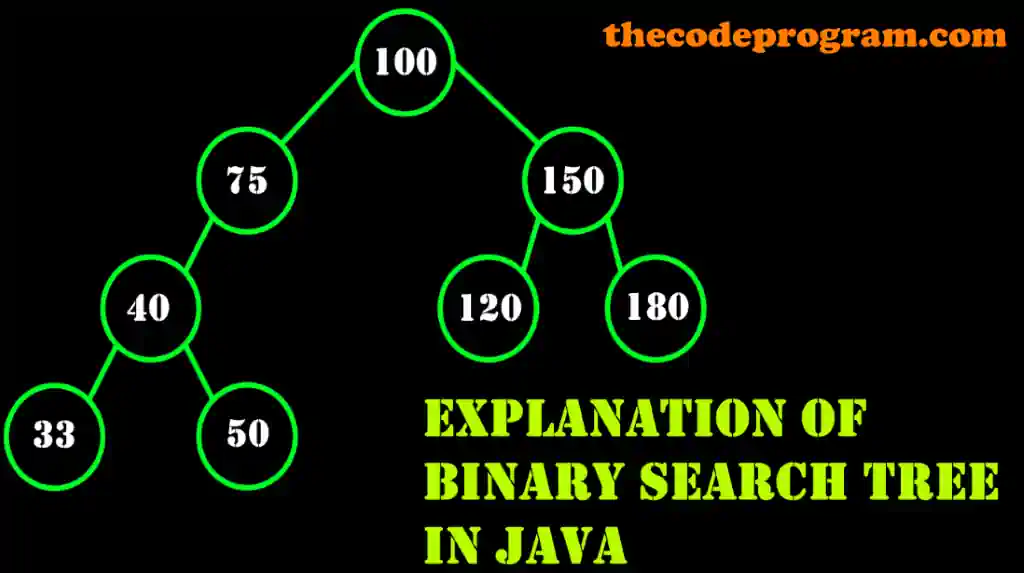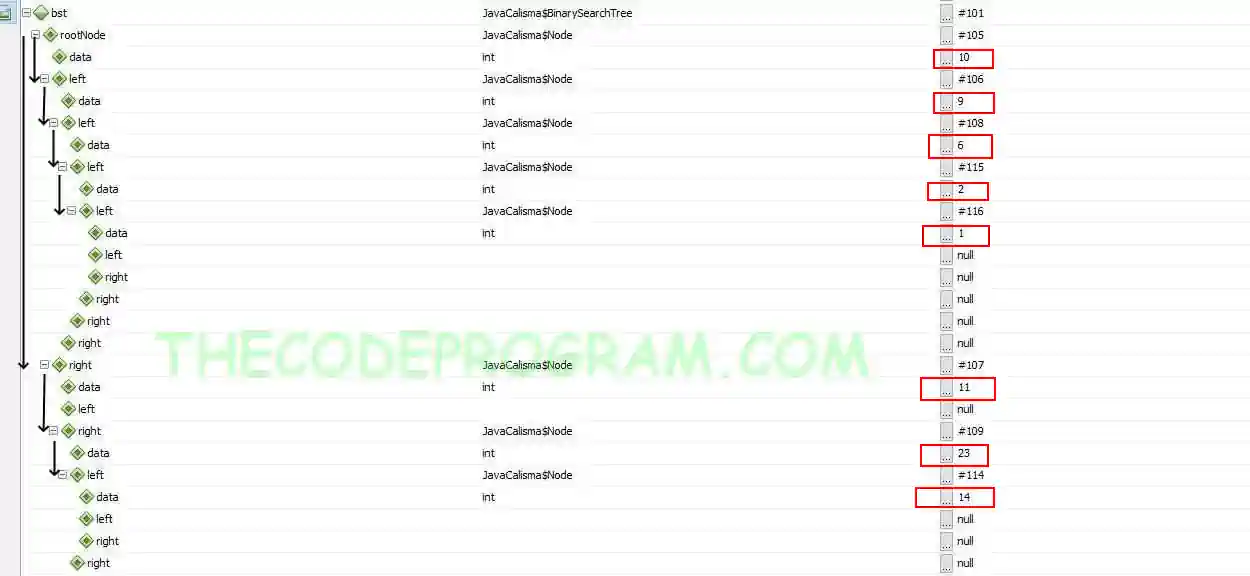## Explanation of Binary Search Tree in Java

Hello everyone, in this article we are going to talk about Binary Search Tree. First we are make a description and then we are going to make an example about Binary Search Tree with Java and Netbeans.

Let's get started.

Firstly What is Binary Search Tree
Binary Search Tree is a data library which based on Nodes. Every node holds the own datas and also keeps its left or right nodes. In here every nodes are also sub Search Trees.

A node Binary Search Tree must have
• The left node which contains nodes with lesser values than current node.
• The right node which contains nodes with greater values than current node.

Now Let's make an example according to above informations.

In this example firstly we are going to create a Node Class which hold the tree with substrees. And we are going to make the searching in this node. This node also keeps its left and right nodes as same types.

Below code block you can see a Binary Search Tree Node Class:
``````
public static class Node{
int data;
Node left = null;
Node right = null;
public Node(int data) { this.data = data; }
}
``````

Now we are ready to make some operations. In here we are going to add new values in this tree. Also we are going to delete the value if existing. And also we are going to find the data in related tree.

So firstly we need to create a BinarySearchTree Class with its rootNode:
``````
public static class BinarySearchTreeStructure{
//first we need to create a root node
Node rootNode;
}
``````

NOTE : All below code blocks which are BinarySearchTree operations will be written in this class.

#### Now let's add some value to our search tree:

We are going to write a recursive method which search the correct location for the data. That location will be empty so we are going to search until find a null data with make the comparisations. Below you can see the code block which make this operations.

``````
//Make public below private method

//This adding method will be recursive
private Node addNode(Node current, int data){
//If there is no added value, create a new node
if(current == null) return new Node(data);
//If added value is exist, make decision to go left or right
//according to data comparisation
if(data < current.data) current.left = addNode(current.left, data);
else if(data > current.data) current.right = addNode(current.right, data);
//if data will not go left or right
else return current;

return current;
}
``````

#### Now Let's find a data in our BinarySearchTree:

To find a data in a BinarySearchTree we are going to use recursive methods again. In here we are going to control the data until find it or until find null data. If we reach the null, it means the search tree does not have the value that we are looking for.

Below code block you can see a required methods. At findNode we have done what we talked about above. We have searched the data at every nodes with comparisation according to BST logic. We go left if data smaller than current node, and we go right if the searching data greater than selected node.

``````
public boolean isNodeExist(int data){ return findNode(rootNode, data); }

private boolean findNode(Node current, int data){
//if incomin node is null returns null
//if income node has the data which we are looking for returns true
if(current == null) return false;
if(data == current.data) return true;

//if we can not find the data it will go left or right according to comparisation
if(data < current.data) return findNode(current.left, data);
else if(data > current.data) return findNode(current.right, data);

return false;
}
``````

#### Lastly lets delete a node in Binary Search Tree.

Firstly we are going to search the data which will be deleted until we find it. This logic is the same with above. We will make comparisations and go left or right according to being smaller or greater than the Node value. Then when we find the data we are ready to delete now.

Now to delete a value we have to take care of three situations:
• If found Node has no sub tree
• If found Node has only one sub tree
• If found Node has both left and right sub trees.
• If there is no sub tree we can remove the found node directly with assigning null.
• If there is only one sub tree we can overwrite sub tree on found Node it has been deleted with securing its subtrees.
• If there is both left and right subtrees we will overwrite the left or right node data to keep nodes.
``````
public void delete(int data){ deleteNode(rootNode, data); }

private Node deleteNode(Node current, int data){
if(current==null) return null;
if(data == current.data){
//deletion process
//if found node has no sub nodes set it as null directly
if(current.left == null && current.right ==null){
return  null;
}

//if node has only one sub node kendisini null yap ve alt nodu buna ata
if(current.right == null) return current.left;
else if(current.left == null) return current.right;

//DO NOT ASSIGN ONE OF NODES HERE, BECAUSE IT WILL REMOVE THE OTHER NODES
if(current.left != null && current.right !=null){
int smallestData = findLeft(current.right);
current.data = smallestData;
current.right = deleteNode(current.right, smallestData);
}
}
else if(data < current.data){
current.left = deleteNode(current.left, data);
}
else if(data > current.data){
current.right = deleteNode(current.right, data);
}
return current;
}
private int findLeft(Node node){
return node.left == null ? node.data : findLeft(node);
}
``````
Above all processes are ready. Now we are going to call these functions. Below we can see the functions on main function.
``````
public static void main(String[] args) {

JavaCalisma.BinarySearchTree bst = new JavaCalisma.BinarySearchTree();
int[] data = {10, 11, 9, 6, 15, 2, 23, 14, 1};

for(int i=0; i<data.length; i++){
}

boolean wasFound = bst.isNodeExist(15);
bst.delete(15);
System.out.println("");
}
``````
Now we can see example output. Below image you can see the debug screen of which taken from netbeans ide.Burak Hamdi TUFAN

10/04/2020

24/06/2020

20/10/2021